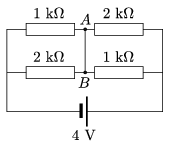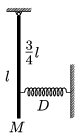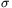Mathematical and Physical Journal
for High Schools
Issued by the MATFUND Foundation
 Already signed up? New to KöMaL?

# KöMaL Problems in Physics, October 2006

Show/hide problems of signs:## Problems with sign 'M'

Deadline expired on November 13, 2006.

M. 272. Measure the work done while a slinky is raised by its uppermost turn. Observing the distance between the turns find the height of the centre of its mass.

(6 pont)

statistics## Problems with sign 'P'

Deadline expired on November 13, 2006.

P. 3913. At which point should the right triangle of sides 3 dm, 4 dm and 5 dm, be suspended if its hypotenuse is vertical. The triangle is made of a piece of thin wire.

(4 pont)

statistics

P. 3914. Find the current in the wire between points A and B.(4 pont)

statistics

P. 3915. The track of motorcycle-race is circular and unbanked. There are two bikers on the road one travels along a path of greater radius than the other. They both lean toward the centre at the same angle. Which one completes the lap first?

(4 pont)

statistics

P. 3916. A small ball is thrown from a height of 1 m, and 2 m away from a vertical wall. The ball bounces on the ground and on the vertical wall totally elastically and then it flies back to its initial position of throwing. Find the initial speed of the ball if it was thrown at an angle of 45o with the horizontal. (Air drag can be neglected).

(5 pont)

statistics

P. 3917. A tram, filled with stones, moves along a on a horizontal straight track. Its total mass with the stones is M. How much work is done while a stone of mass m is thrown out of the tram with a horizontal speed of v with respect to the tram if it is thrown backwards or if it is thrown forward. Find the kinetic energy of the stone in both cases.

(5 pont)

statistics

P. 3918. A beam of mass M and of length l is attached to a pivot hinge where the friction is negligible as shown in the figure. At 3 of the beam a spring is attached to it and its other end is connected to the wall. (The spring has a spring constant D and it can both pull an push.) Find the period of the beam for small displacements.(5 pont)

statistics

P. 3919. There is a vertical cylinder filled with a sample of ideal gas in a thermostat. A horizontal piston which can move easily, splits the cylinder into two parts. The volume of the upper part is 4 times as much as the volume of the part below. If the cylinder is placed to a thermostat at a temperature of 400 K the ratio of the volumes of the upper and lower parts will be 3:1. Find the ratio of the number of molecules of the gas in the two parts of the cylinder.

(4 pont)

statistics

P. 3920. A large vertical insulating sheet is charged uniformly. The surface charge density is. A small neutral body of mass m is suspended on a long insulating thread of length l next to the sheet. Then the small body is given a charge of q, and left alone.

a) Find the greatest angle between the thread and the vertical.

b) Find the greatest speed of the small body.

c) Find the period of the motion. Data: l=1 m, m=10-3 kg, q=10-8 C,=10-6 C/m2.

(5 pont)

statistics

P. 3921. An electron revolves along circular path in uniform magnetic field of 1 T. Find the radius of the circle. The kinetic energy of the electron is a) 5.1 keV, b) 510 keV, c) 51 MeV.

(5 pont)

statistics

P. 3922. How much energy is needed to split the nucleus of ainto four alpha-particles.

(4 pont)

statistics